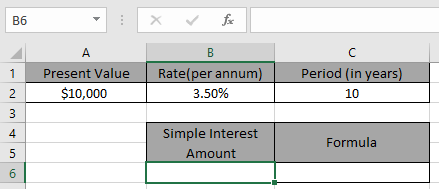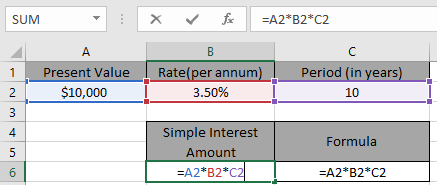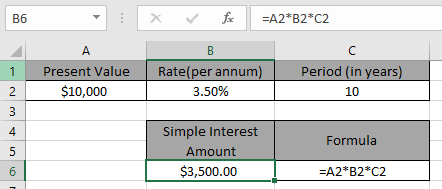# How to calculate Simple interest formula in Excel

In this article, we will learn how to use Simple interest formula in Excel.

Calculate the simple interest amount given the present or principal amount, rate in annum & period in years.
Syntax:

=PA * rate * period

PA : principal amount
Rate : Rate per annum
Period : Period in years

Let’s understand this function using an example.
Here we have a data set and to get Simple interest(SI) amountWe need to find the simple interest amount for the dateset.

Use the formula to get the simple interest amount

=A2 * B2 * C2Press EnterAs you can see the simple interest amount for the dataset is \$ 3500.

Note: Remember to keep the data in term of years.

Hope you understood how to get the simple interest amount in Excel. Explore more articles on Excel statistical function here. Please feel free to state your query or feedback for the above article.

Related Articles:

Excel IPMT Function

How to use Compound Interest Function in Excel

Popular Articles:

50 Excel Shortcuts to Increase Your Productivity

How to use the VLOOKUP Function in Excel

How to use the COUNTIF function in Excel

How to use the SUMIF Function in Excel

Terms and Conditions of use

The applications/code on this site are distributed as is and without warranties or liability. In no event shall the owner of the copyrights, or the authors of the applications/code be liable for any loss of profit, any problems or any damage resulting from the use or evaluation of the applications/code.